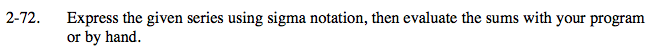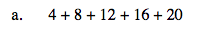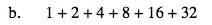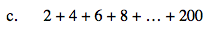### Home > PC > Chapter 2 > Lesson 2.3.1 > Problem2-72

2-72.
1. Express the given series using sigma notation, then evaluate the sums with your program or by hand. Homework Help ✎

1. 4 + 8 + 12 + 16 + 20

2. 1 + 2 + 4 + 8 + 16 + 32

3. 2 + 4 + 6 + 8 + … + 200All of the numbers in the series are multiples of 4.The numbers in this series are all powers of 2.
It may be easier to start with an index of k = 0 instead of k = 1.All of the numbers in the series are multiples of 2.